# Quiz 13: Monetary Policy: Conventional and Unconventional

Assume there is \$120 billion of cash and half of this cash is held in bank as required reserve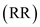. It means the required reserve is \$60 billion (\$120 billion/2). The size of money supply can be calculated by using the following formula: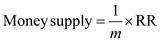……(1) To find the size of money supply at 10 percent required reserve ratio, substitute the respective values in Equation (1):Hence, the size of the economy will be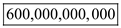. To find the size of money supply at 12.5 percent required reserve ratio, substitute the respective values in Equation (1):Hence, the size of the economy will be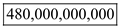. To find the size of money supply at 16.67 percent required reserve ratio, substitute the respective values in Equation (1):Hence, the size of the economy will be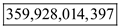.